## Resource of the Week: How to do written multiplication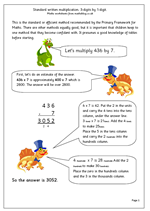Some of our most popular resouces are to do with written methods of addition, subtraction, multiplication and division. This is just one of  our worksheets that show how to use the standard or efficient method to multiply a 3-digit number by a single digit. There are other methods equally good, but it is important that children keep to one method that they become confident with. It presumes a good knowledge of tables before starting.

For example: 436 x 7.

First, do an estimate of the answer.
436 x 7 is approximately 400 x 7 which is 2800. The answer will be over 2800.
Then set the sum out in a vertical layout:
436
x 7

6 x 7 is 42. Put the 2 in the units and carry the 4 tens into the tens column, under the answer line.
Then multiply the tens.
3 (tens)  x 7 is 21(tens). Add the 4 (tens).  to make 25(tens).
Place the 5 in the tens column and carry the 2 (hundreds) into the hundreds column.

Finally the hundreds.
4 (hundreds) x 7 is 28 (hundreds). Add the 2 (hundreds) to make 30 (hundreds).
Place the zero in the hundreds column and the 3 in the thousands column.
A clearer layout of this is available on the worksheet explanation.

Further written multiplication worksheets can be found in the Year 5 and Year 6 Calculations sections.

Standard written multiplication: 3-digits by 1-digit

## Year 3 Mental Arithmetic: Sets 39 and 40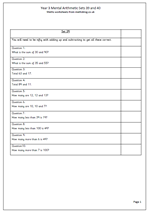We are well under way with our sets of Year 3 Mental Arithmetic worksheets, with sets 39 and 40 coming up this week. Remember these pages can be used in a number of different ways. The questions can be read out and answered orally, or the answers can be written down on paper. The question sheets can be printed out and used whilst reading the questions out loud as an extra help.

This week the questions are all on addition and subtraction and children who are good at maths will use a variety of different approaches to answer them.

For example: ‘How many are 12, 12 and 13?

Another approach is to double 12 and add 13 to the answer.

A third approach is to multiply 12 by 3 and add 1. This is probably the quickest method if children know ‘times tables’ up to 12 x 12.

Whichever approach is used, one of the most valuable aspects of giving mental arithmetic questions is to follow it up by asking what approach was used, and suggesting methods which might be more efficient.

Year 3 mental arithmetic (sets 39 and 40)

## Problem Solving Worksheet for Year 4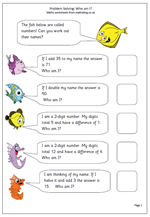There are only 5 questions on this page but some children will find it quite difficult. The main reason for this is that they will have to work back from the answer to find the starting number. This will often mean reversing the operations, subtracting where it says add, halving where it says double etc.

This is a god test of children’s knowledge of the relationships between addition and subtraction, doubling and halving and multiplication and division.

Two of the harder questions come at the end, because they involve carrying out two processes to reach the answer. For example:

‘I am a 2-digit number. My digits total 10 and have a difference of 2. Who am I?’

This involves finding 2 digits which have a total of 10 (2 and 8, 3 and 7 etc) and then checking that the difference between the two digits is 2.

6 and 4 will solve the problem so there are two possible answers: 46 or 64.

Solve problems: Who am I?

## Resource of the week: reflective symetry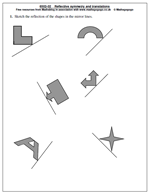Reflective symmetry seems to be a very popular topic for the Key Stage 2 Maths SAT Papers and it is not easy to find examples of the kind of question which is often asked. There are several mathematical terms to do with symmetry that children need to be familiar with. These include:

Mirror line,  line of symmetry,  line symmetry,  symmetrical,  reflect,  reflection,  translation, axis of symmetry, reflective symmetry.

Also they should be able to test for symmetry using a mirror and by folding.

Children should be able to sketch the reflection of a simple shape in a mirror line where none or only some of the edges of the shape are parallel or perpendicular to the mirror line.

This might seem easy, but actuallyoften proves to be problematic to many children. A small mirror is a great help with this and children are supplied with one in their SAT tests if such a question comes up.

Here we have the second of a pair of worksheets which looks at sketching the reflection of shapes in the mirror lines.

Year 6 maths worksheet: Reflective symmetry

## Year 3 Mental Arithmetic: Counting on and back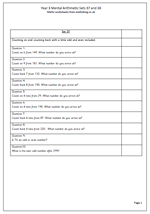An ability to calculate mentally lies at the heart of being successful with number work. Mental methods need to be emphasised and practised on a regular basis and these year 3 mental arithmetic pages can certainly help towards this.

These two sets of ten questions concentrate on counting on and back in single figures or whole tens. Children will often use their fingers to count on or back but it does help if they can begin to know these facts off by heart. For example counting back 7 from a number with a 2 in the units will result in an answer with 5 in the units. By the end of year 3 children should also be confident with counting on and back in whole tens from any 2-digit number.

Year 3 Mental arithmetic (Sets 37 and 38)

## Pancake day: maths worksheet on ratio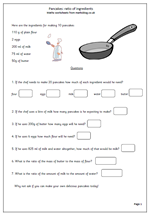Pancake Day is usually known as Shrove Tuesday and it the last day before the period known as Lent in the Christian calendar. The idea is that Shrove Tuesday is the last chance to use up foods that you are not allowed during Lent, a time of abstinence and giving things up.
Pancakes  are eaten on this day because they contain butter, eggs and fat which are not allowed during Lent.
This maths worksheets takes a look at the ingredients needed to make pancakes and then asks some pretty tricky questions involving ratio, so it is probably best suited to Year 6 children.
It is not easy to find ratio worksheets, but recipes are an ideal subject.
Thanks to urbrainy.com for letting me use this page from their site and they have many more great maths worksheets on special occasions as well as thousands of other maths worksheets.

This page can be found in the year 6 Understanding Number category.

Pancake Day: ratio

## Valentine’s Day maths worksheet: Subtraction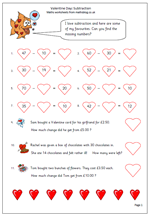Sometimes it’s nice to have a change and special occasions such as Valentine’s Day allows us to do this. Here we have a maths worksheet suitable for Year 3 children with a Valentine Day’s theme. It is a subtraction page but all the missing numbers have to be put into the special Valentine hearts. There are also a couple of Valentine word problems.

The easiest way to do most of these subtractions is by adding on from the smaller number, once again showing the special relationship between addition and subtraction.

Thanks to urbrainy.com for letting me use this page from their vast supply of worksheets, including more on Valentine’s Day.

Valentine subtraction

## Maths SAT Paper 2011 Paper B Questions 8 and 9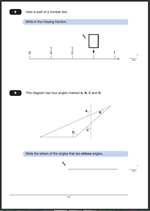Here are two more questions from the Maths SAT Paper 2011 Paper B.

The answer to Question 8.   3/4
One mark awarded for a correct answer.
Equivalent fractions accepted as is 0.75.
Suggested method:
This question is another reading a number line problem and if children can count in quarters it is an easy mark. Firstly, it has to be recognised that the number line is showing quarters (1/2 is equivalent to 2/4 and 1 is equivalent to 4/4) making the missing number 3/4.

The answer to Question 9. A and D.
One mark awarded for a correct answer.
The letters can be given in either order. Both need to be given with no incorrect angles added.
Suggested method:
Answering this question correctly depends on 3 things:
Firstly, a recognition of the conventions for labelling angles, using the arc.
Secondly, a knowledge that an obtuse angle is larger than 90? but less than 180?.
If either of these are unknown it becomes purely a guessing game. (Some children do think that the angle depends on the length of the lines rather than a measure of turn.)

Thirdly, if these are known, then the obtuse angles still need to be recognised. One way to do this is to slide a right angle (eg a corner of a piece of paper) into the angle to see whether it is smaller or larger.

Why not visit ks2-maths-sats.co.uk for free SATs papers and a great SAT revision programme?

Questions 8 and 9 from SAT Paper B 2011

Questions 8 and 9 answers and suggested method 2011 Paper B

## Missing digits in subtraction sentences: year 3 maths worksheet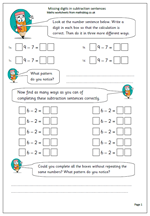By the end of year 3 children are expected to be able to subtract a single digit from any 2-digit number. Knowing the patterns involved can make this much easier and this worksheet looks at these patterns.

The number sentence has three missing digits and children are asked to write a digit in each box so that the calculation is correct. There is a limited number of possible correct answers and the second part of the page looks at finding all of them. This is a fairly straightforward page as there is no adjusting or decomposition involved. Look for a logical, well ordered way of working for the second part of the question so that all possible answers are found.

Missing digits in subtraction

## Year 3 mental arithmetic: Sets 35 and 36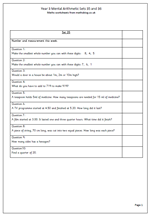Exactly half way through the school year with the publication of sets 35 and 36 mental arithmetic for Year 3. This is quite a mixed bag of questions covering number and measurement.

Two of the questions in each set look at time, which is often a problem for children. These two questions look at time intervals between two events. For example:

‘A TV programme starts at 4.50 and ends at 5.20. How long does it last?’

This presumes that knowledge of 60 minutes in an hour is known and the best way to proceed is to add on to the next whole hour (10 minutes) and then add on the extra minutes (20 minutes) to get 30 minutes.

These questions can be read out loud and children answer on paper, or they can just call out the answers, or show them on digit cards etc. Alternatively they can be given the question sheet to look at and record answers.

Year 3 Mental Arithmetic (sets 35 and 36)